# Selina Solutions Concise Mathematics Class 6 Chapter 17: Idea of Speed, Distance and Time Exercise 17(B)

Selina Solutions Concise Mathematics Class 6 Chapter 17 Idea of Speed, Distance and Time Exercise 17(B) explains all the basic terms in an understandable language, which are important from the exam perspective. The solutions PDF contain multiple tricks to solve the difficult problems effortlessly. In-depth practice of these solutions helps students boost exam preparation, along with scoring more marks in the annual examination. Those who aspire to become experts in Mathematics, are suggested to follow Selina Solutions Concise Mathematics Class 6 Chapter 17 Idea of Speed, Distance and Time Exercise 17(B) PDF, from the below mentioned links.

## Selina Solutions Concise Mathematics Class 6 Chapter 17: Idea of Speed, Distance and Time Exercise 17(B) Download PDF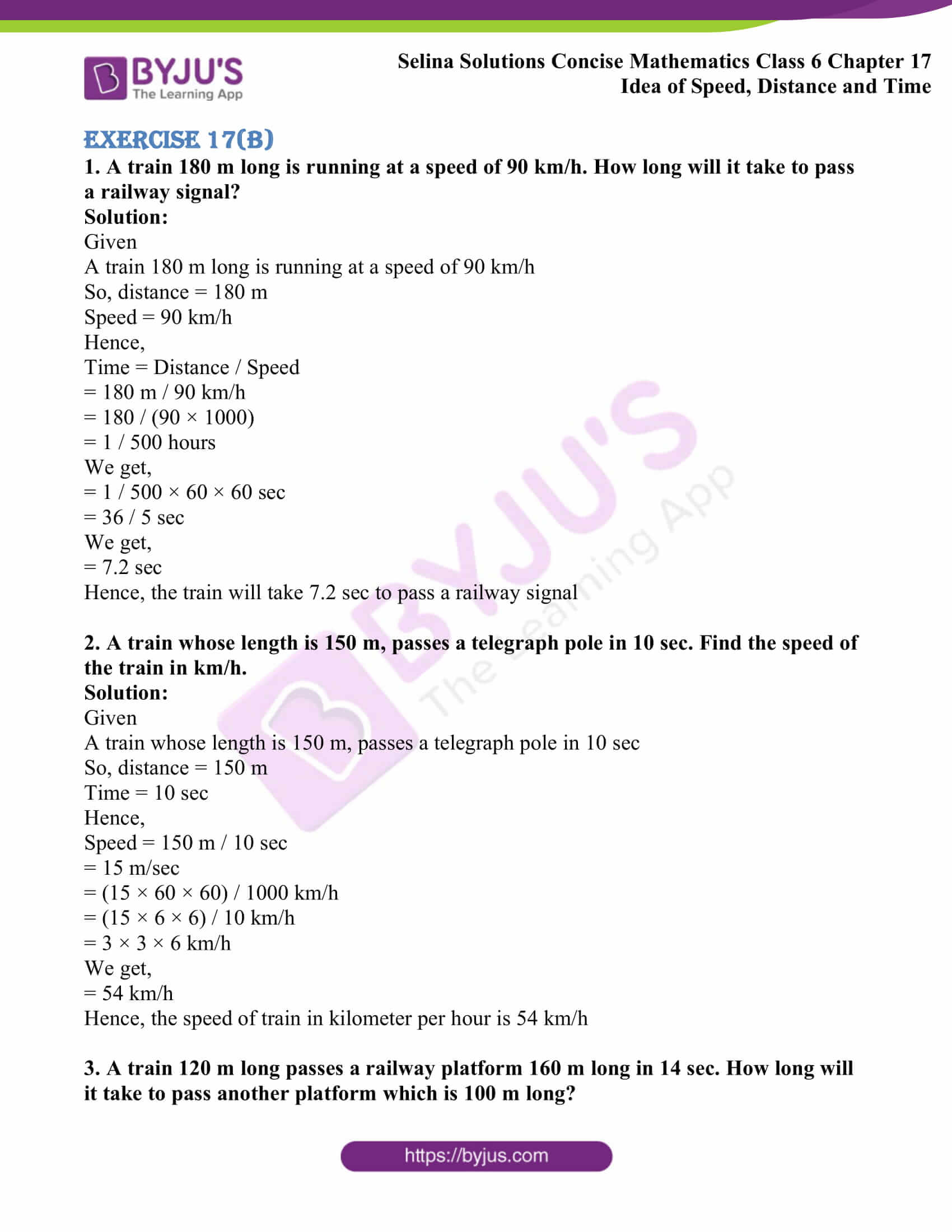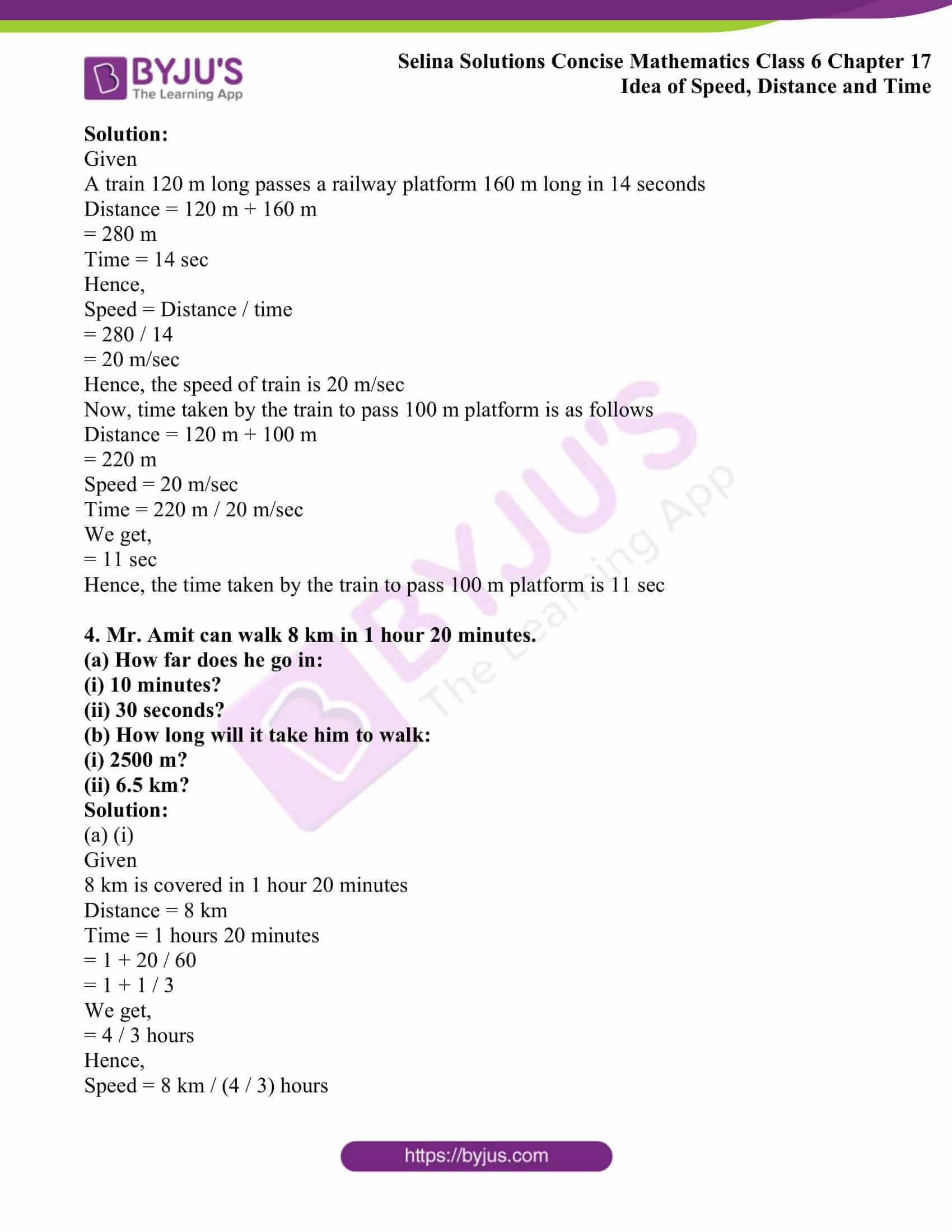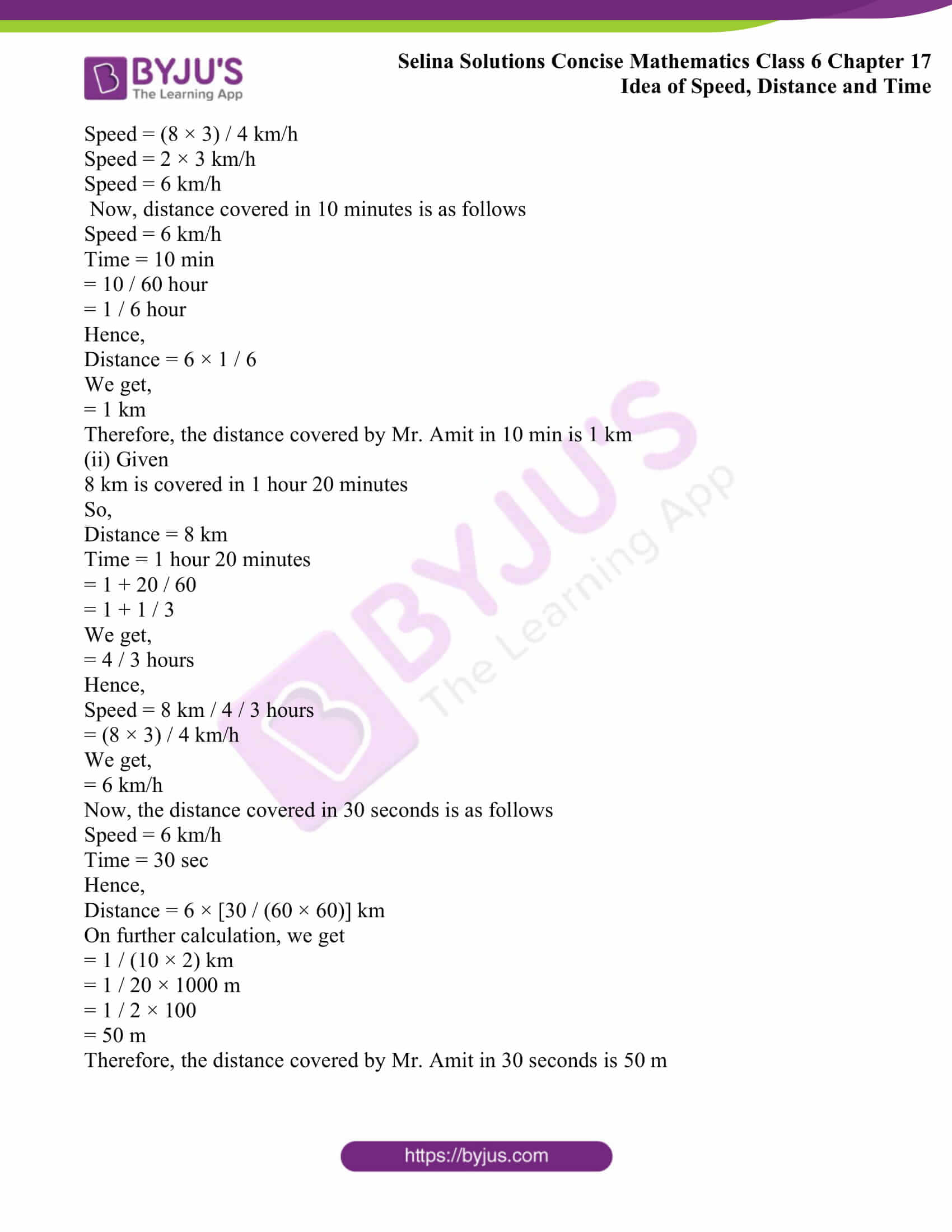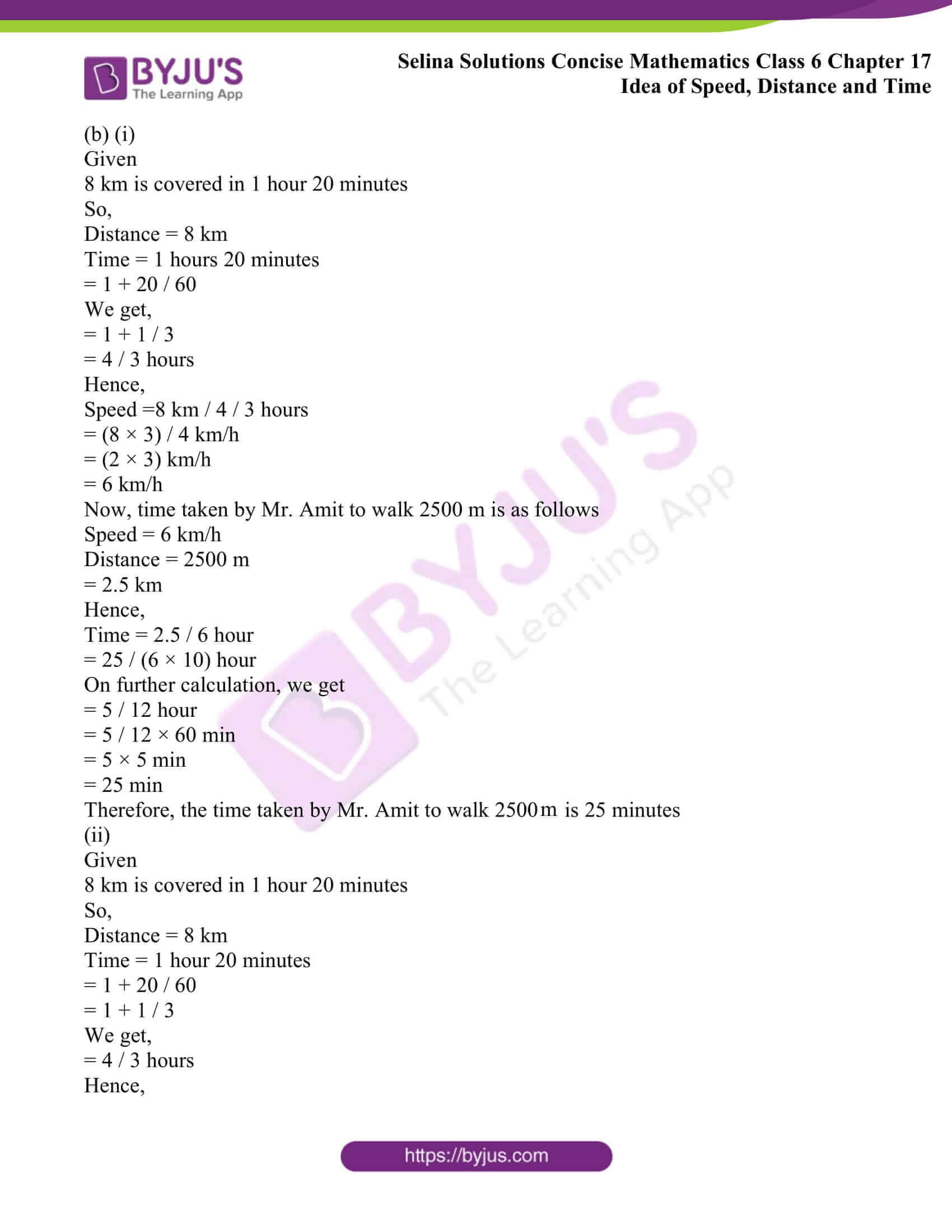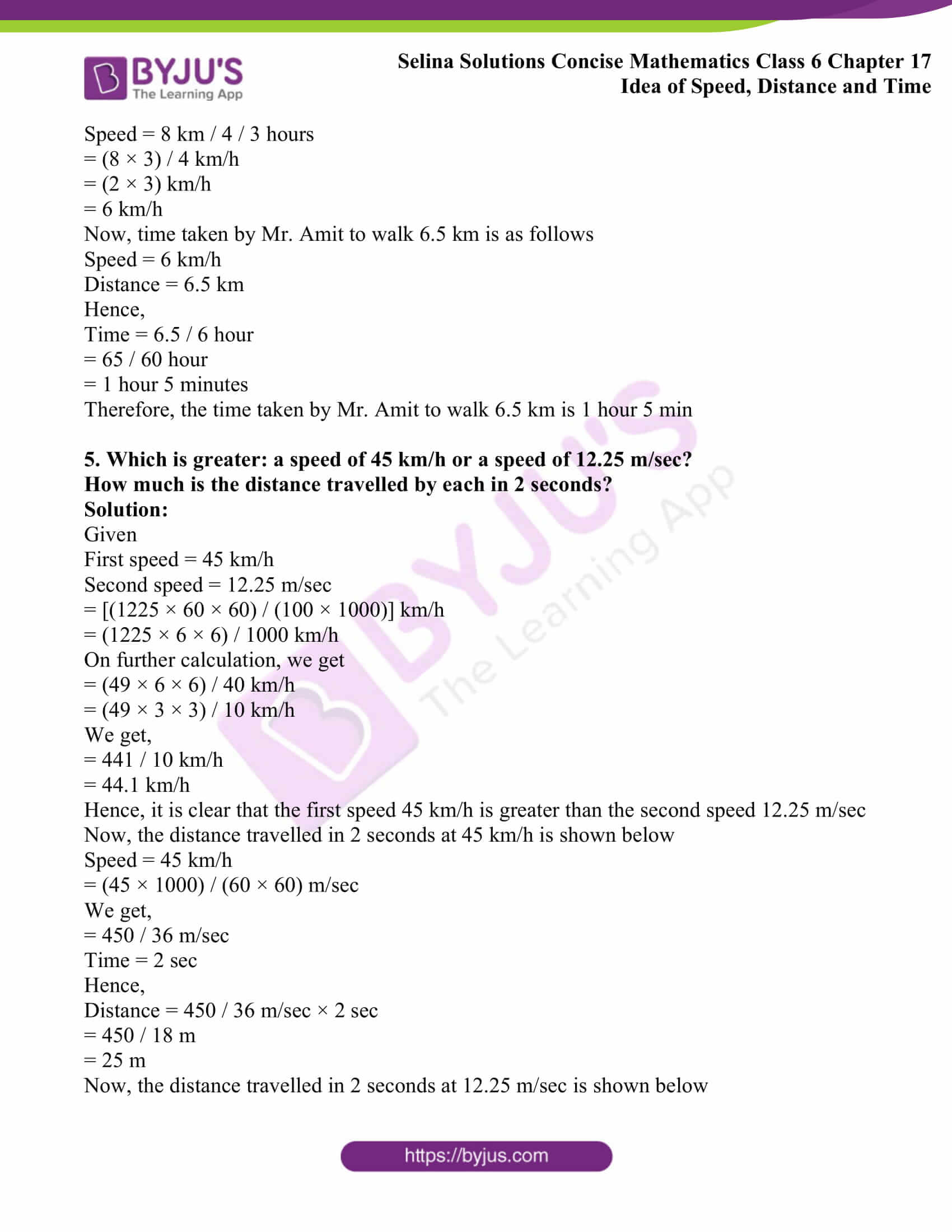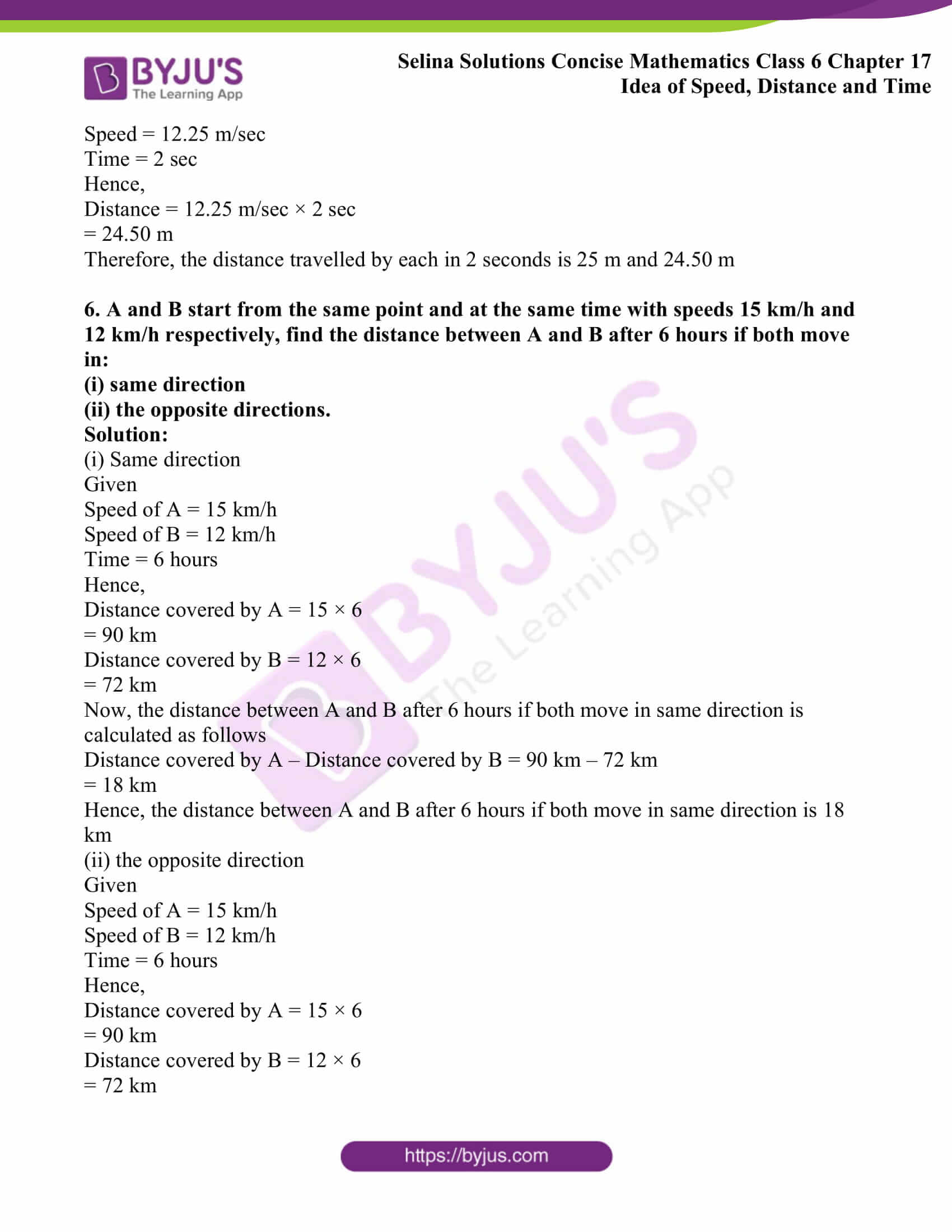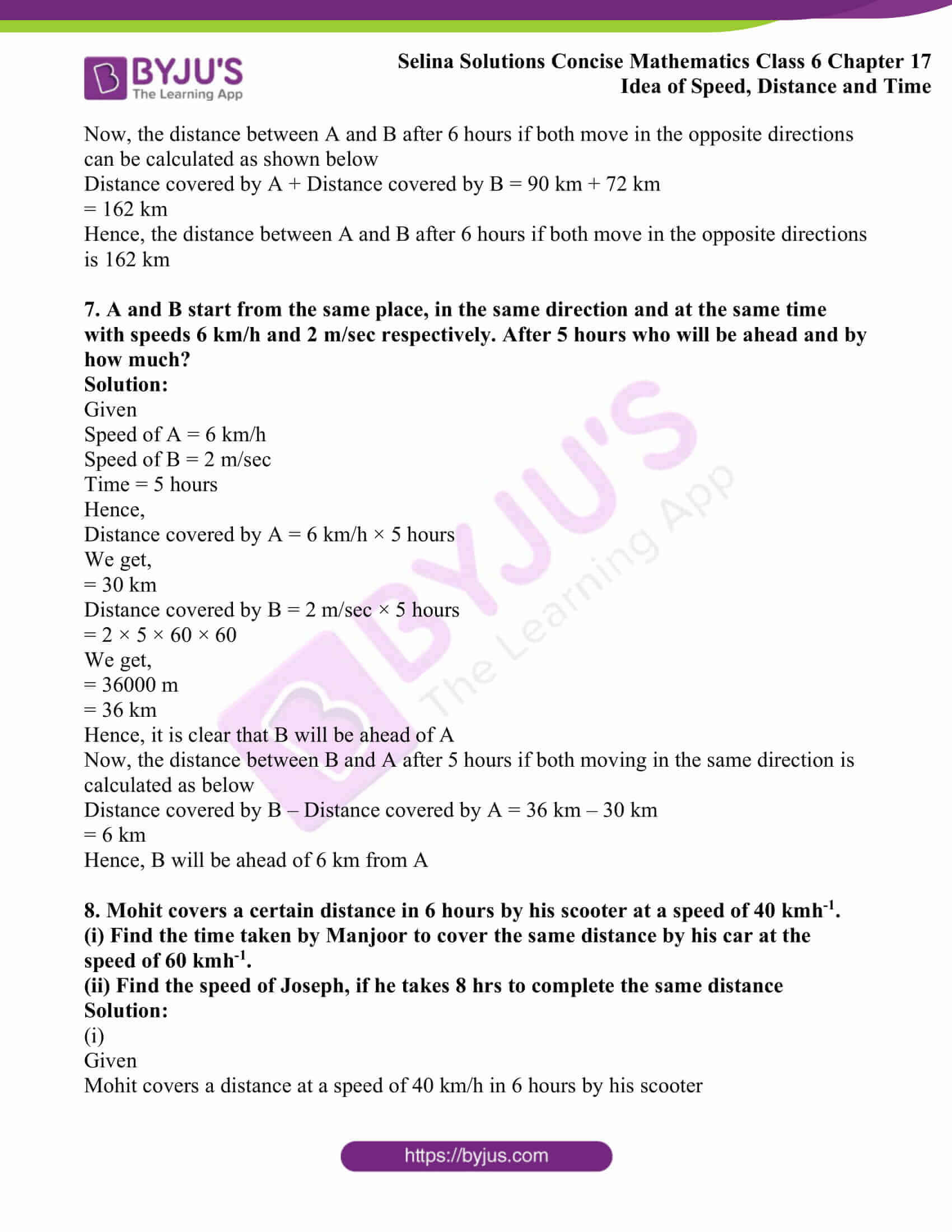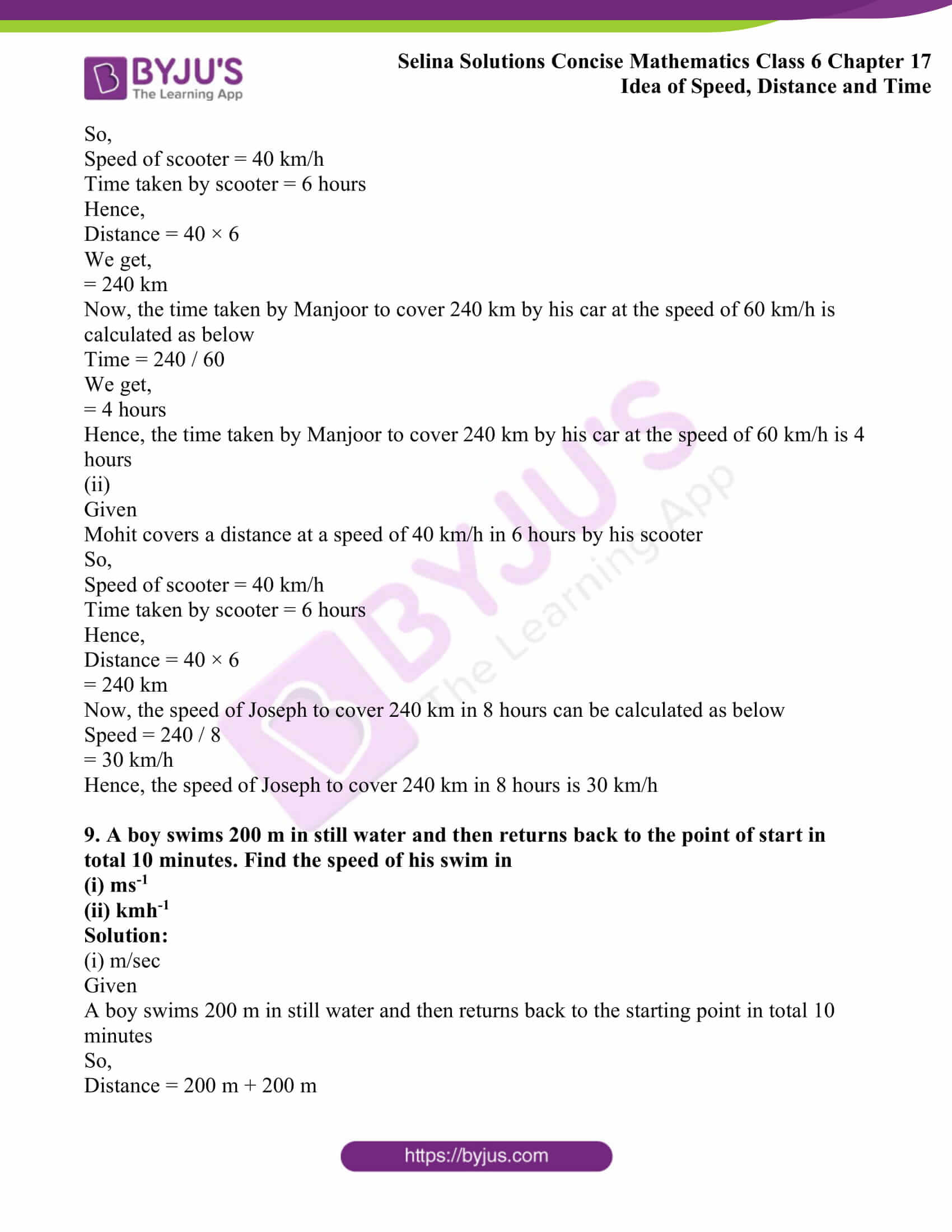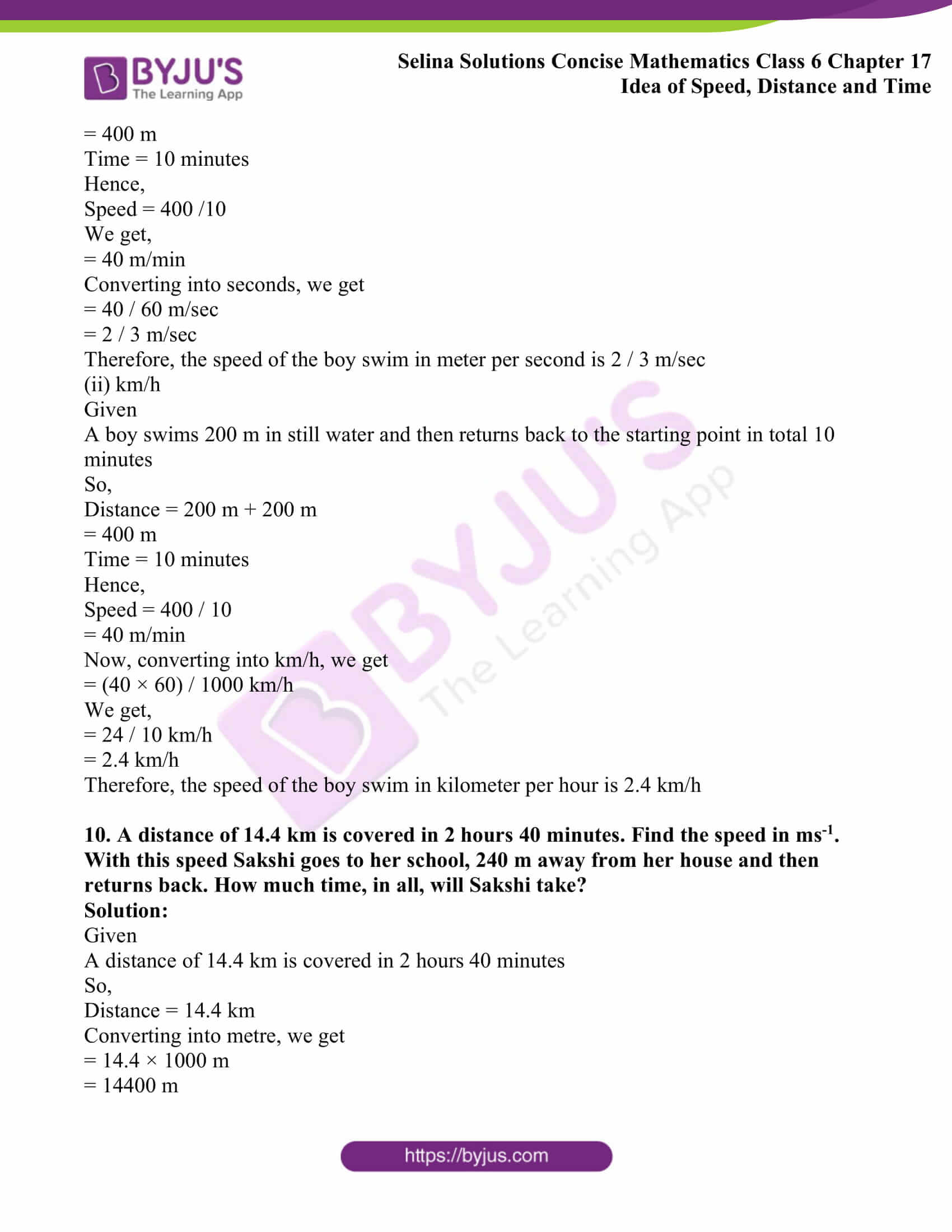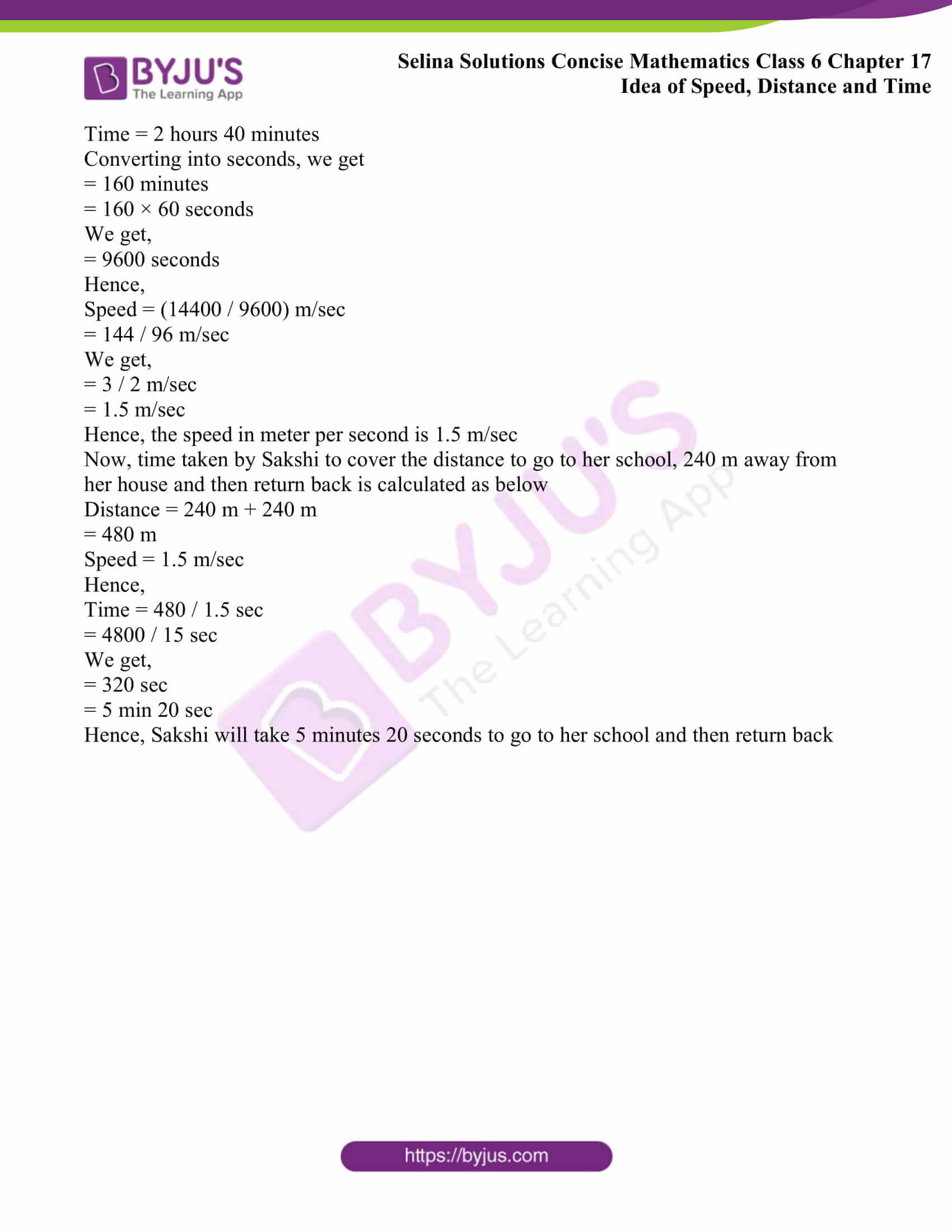### Access another exercise of Selina Solutions Concise Mathematics Class 6 Chapter 17: Idea of Speed, Distance and Time

Exercise 17(A) Solutions

### Access Selina Solutions Concise Mathematics Class 6 Chapter 17: Idea of Speed, Distance and Time Exercise 17(B)

#### Exercise 17(B)

1. A train 180 m long is running at a speed of 90 km/h. How long will it take to pass a railway signal?

Solution:

Given

A train 180 m long is running at a speed of 90 km/h

So, distance = 180 m

Speed = 90 km/h

Hence,

Time = Distance / Speed

= 180 m / 90 km/h

= 180 / (90 × 1000)

= 1 / 500 hours

We get,

= 1 / 500 × 60 × 60 sec

= 36 / 5 sec

We get,

= 7.2 sec

Hence, the train will take 7.2 sec to pass a railway signal

2. A train whose length is 150 m, passes a telegraph pole in 10 sec. Find the speed of the train in km/h.

Solution:

Given

A train whose length is 150 m, passes a telegraph pole in 10 sec

So, distance = 150 m

Time = 10 sec

Hence,

Speed = 150 m / 10 sec

= 15 m/sec

= (15 × 60 × 60) / 1000 km/h

= (15 × 6 × 6) / 10 km/h

= 3 × 3 × 6 km/h

We get,

= 54 km/h

Hence, the speed of train in kilometer per hour is 54 km/h

3. A train 120 m long passes a railway platform 160 m long in 14 sec. How long will it take to pass another platform which is 100 m long?

Solution:

Given

A train 120 m long passes a railway platform 160 m long in 14 seconds

Distance = 120 m + 160 m

= 280 m

Time = 14 sec

Hence,

Speed = Distance / time

= 280 / 14

= 20 m/sec

Hence, the speed of train is 20 m/sec

Now, time taken by the train to pass 100 m platform is as follows

Distance = 120 m + 100 m

= 220 m

Speed = 20 m/sec

Time = 220 m / 20 m/sec

We get,

= 11 sec

Hence, the time taken by the train to pass 100 m platform is 11 sec

4. Mr. Amit can walk 8 km in 1 hour 20 minutes.

(a) How far does he go in:

(i) 10 minutes?

(ii) 30 seconds?

(b) How long will it take him to walk:

(i) 2500 m?

(ii) 6.5 km?

Solution:

(a) (i)

Given

8 km is covered in 1 hour 20 minutes

Distance = 8 km

Time = 1 hour 20 minutes

= 1 + 20 / 60

= 1 + 1 / 3

We get,

= 4 / 3 hours

Hence,

Speed = 8 km / (4 / 3) hours

Speed = (8 × 3) / 4 km/h

Speed = 2 × 3 km/h

Speed = 6 km/h

Now, distance covered in 10 minutes is as follows

Speed = 6 km/h

Time = 10 min

= 10 / 60 hour

= 1 / 6 hour

Hence,

Distance = 6 × 1 / 6

We get,

= 1 km

Therefore, the distance covered by Mr. Amit in 10 min is 1 km

(ii) Given

8 km is covered in 1 hour 20 minutes

So,

Distance = 8 km

Time = 1 hour 20 minutes

= 1 + 20 / 60

= 1 + 1 / 3

We get,

= 4 / 3 hours

Hence,

Speed = 8 km / 4 / 3 hours

= (8 × 3) / 4 km/h

We get,

= 6 km/h

Now, the distance covered in 30 seconds is as follows

Speed = 6 km/h

Time = 30 sec

Hence,

Distance = 6 × [30 / (60 × 60)] km

On further calculation, we get

= 1 / (10 × 2) km

= 1 / 20 × 1000 m

= 1 / 2 × 100

= 50 m

Therefore, the distance covered by Mr. Amit in 30 seconds is 50 m

(b) (i)

Given

8 km is covered in 1 hour 20 minutes

So,

Distance = 8 km

Time = 1 hour 20 minutes

= 1 + 20 / 60

We get,

= 1 + 1 / 3

= 4 / 3 hours

Hence,

Speed =8 km / 4 / 3 hours

= (8 × 3) / 4 km/h

= (2 × 3) km/h

= 6 km/h

Now, time taken by Mr. Amit to walk 2500 m is as follows

Speed = 6 km/h

Distance = 2500 m

= 2.5 km

Hence,

Time = 2.5 / 6 hour

= 25 / (6 × 10) hour

On further calculation, we get

= 5 / 12 hour

= 5 / 12 × 60 min

= 5 × 5 min

= 25 min

Therefore, the time taken by Mr. Amit to walk 2500 m is 25 minutes

(ii)

Given

8 km is covered in 1 hour 20 minutes

So,

Distance = 8 km

Time = 1 hour 20 minutes

= 1 + 20 / 60

= 1 + 1 / 3

We get,

= 4 / 3 hours

Hence,

Speed = 8 km / 4 / 3 hours

= (8 × 3) / 4 km/h

= (2 × 3) km/h

= 6 km/h

Now, time taken by Mr. Amit to walk 6.5 km is as follows

Speed = 6 km/h

Distance = 6.5 km

Hence,

Time = 6.5 / 6 hour

= 65 / 60 hour

= 1 hour 5 minutes

Therefore, the time taken by Mr. Amit to walk 6.5 km is 1 hour 5 min

5. Which is greater: a speed of 45 km/h or a speed of 12.25 m/sec?

How much is the distance travelled by each in 2 seconds?

Solution:

Given

First speed = 45 km/h

Second speed = 12.25 m/sec

= [(1225 × 60 × 60) / (100 × 1000)] km/h

= (1225 × 6 × 6) / 1000 km/h

On further calculation, we get

= (49 × 6 × 6) / 40 km/h

= (49 × 3 × 3) / 10 km/h

We get,

= 441 / 10 km/h

= 44.1 km/h

Hence, it is clear that the first speed 45 km/h is greater than the second speed 12.25 m/sec

Now, the distance travelled in 2 seconds at 45 km/h is shown below

Speed = 45 km/h

= (45 × 1000) / (60 × 60) m/sec

We get,

= 450 / 36 m/sec

Time = 2 sec

Hence,

Distance = 450 / 36 m/sec × 2 sec

= 450 / 18 m

= 25 m

Now, the distance travelled in 2 seconds at 12.25 m/sec is shown below

Speed = 12.25 m/sec

Time = 2 sec

Hence,

Distance = 12.25 m/sec × 2 sec

= 24.50 m

Therefore, the distance travelled by each in 2 seconds is 25 m and 24.50 m

6. A and B start from the same point and at the same time with speeds 15 km/h and 12 km/h respectively, find the distance between A and B after 6 hours if both move in:

(i) same direction

(ii) the opposite directions.

Solution:

(i) Same direction

Given

Speed of A = 15 km/h

Speed of B = 12 km/h

Time = 6 hours

Hence,

Distance covered by A = 15 × 6

= 90 km

Distance covered by B = 12 × 6

= 72 km

Now, the distance between A and B after 6 hours if both move in same direction is calculated as follows

Distance covered by A – Distance covered by B = 90 km – 72 km

= 18 km

Hence, the distance between A and B after 6 hours if both move in same direction is 18 km

(ii) the opposite direction

Given

Speed of A = 15 km/h

Speed of B = 12 km/h

Time = 6 hours

Hence,

Distance covered by A = 15 × 6

= 90 km

Distance covered by B = 12 × 6

= 72 km

Now, the distance between A and B after 6 hours if both move in the opposite directions can be calculated as shown below

Distance covered by A + Distance covered by B = 90 km + 72 km

= 162 km

Hence, the distance between A and B after 6 hours if both move in the opposite directions is 162 km

7. A and B start from the same place, in the same direction and at the same time with speeds 6 km/h and 2 m/sec respectively. After 5 hours who will be ahead and by how much?

Solution:

Given

Speed of A = 6 km/h

Speed of B = 2 m/sec

Time = 5 hours

Hence,

Distance covered by A = 6 km/h × 5 hours

We get,

= 30 km

Distance covered by B = 2 m/sec × 5 hours

= 2 × 5 × 60 × 60

We get,

= 36000 m

= 36 km

Hence, it is clear that B will be ahead of A

Now, the distance between B and A after 5 hours if both moving in the same direction is calculated as below

Distance covered by B – Distance covered by A = 36 km – 30 km

= 6 km

Hence, B will be ahead of 6 km from A

8. Mohit covers a certain distance in 6 hours by his scooter at a speed of 40 kmh-1.

(i) Find the time taken by Manjoor to cover the same distance by his car at the speed of 60 kmh-1.

(ii) Find the speed of Joseph, if he takes 8 hrs to complete the same distance

Solution:

(i)

Given

Mohit covers a distance at a speed of 40 km/h in 6 hours by his scooter

So,

Speed of scooter = 40 km/h

Time taken by scooter = 6 hours

Hence,

Distance = 40 × 6

We get,

= 240 km

Now, the time taken by Manjoor to cover 240 km by his car at the speed of 60 km/h is calculated as below

Time = 240 / 60

We get,

= 4 hours

Hence, the time taken by Manjoor to cover 240 km by his car at the speed of 60 km/h is 4 hours

(ii)

Given

Mohit covers a distance at a speed of 40 km/h in 6 hours by his scooter

So,

Speed of scooter = 40 km/h

Time taken by scooter = 6 hours

Hence,

Distance = 40 × 6

= 240 km

Now, the speed of Joseph to cover 240 km in 8 hours can be calculated as below

Speed = 240 / 8

= 30 km/h

Hence, the speed of Joseph to cover 240 km in 8 hours is 30 km/h

9. A boy swims 200 m in still water and then returns back to the point of start in total 10 minutes. Find the speed of his swim in

(i) ms-1

(ii) kmh-1

Solution:

(i) m/sec

Given

A boy swims 200 m in still water and then returns back to the starting point in total 10 minutes

So,

Distance = 200 m + 200 m

= 400 m

Time = 10 minutes

Hence,

Speed = 400 /10

We get,

= 40 m/min

Converting into seconds, we get

= 40 / 60 m/sec

= 2 / 3 m/sec

Therefore, the speed of the boy swim in meter per second is 2 / 3 m/sec

(ii) km/h

Given

A boy swims 200 m in still water and then returns back to the starting point in total 10 minutes

So,

Distance = 200 m + 200 m

= 400 m

Time = 10 minutes

Hence,

Speed = 400 / 10

= 40 m/min

Now, converting into km/h, we get

= (40 × 60) / 1000 km/h

We get,

= 24 / 10 km/h

= 2.4 km/h

Therefore, the speed of the boy swim in kilometer per hour is 2.4 km/h

10. A distance of 14.4 km is covered in 2 hours 40 minutes. Find the speed in ms-1. With this speed Sakshi goes to her school, 240 m away from her house and then returns back. How much time, in all, will Sakshi take?

Solution:

Given

A distance of 14.4 km is covered in 2 hours 40 minutes

So,

Distance = 14.4 km

Converting into metre, we get

= 14.4 × 1000 m

= 14400 m

Time = 2 hours 40 minutes

Converting into seconds, we get

= 160 minutes

= 160 × 60 seconds

We get,

= 9600 seconds

Hence,

Speed = (14400 / 9600) m/sec

= 144 / 96 m/sec

We get,

= 3 / 2 m/sec

= 1.5 m/sec

Hence, the speed in meter per second is 1.5 m/sec

Now, time taken by Sakshi to cover the distance to go to her school, 240 m away from her house and then return back is calculated as below

Distance = 240 m + 240 m

= 480 m

Speed = 1.5 m/sec

Hence,

Time = 480 / 1.5 sec

= 4800 / 15 sec

We get,

= 320 sec

= 5 min 20 sec

Hence, Sakshi will take 5 minutes 20 seconds to go to her school and then return back